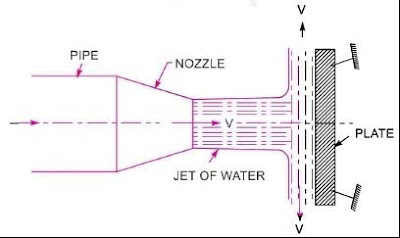# Force exerted on the jet on vertical plate - Ishwaranand

## Force exerted on the jet toward a stationary vertical plate

### IntroductionImage Source ~ Crafted With ©Ishwaranand - 2020 ~ Image by ©Ishwaranand

Fig,  Force exerted by the jet toward a vertical plate

### Force exerted on the jet toward a stationary vertical plate

Let.
v = Velocity of the jet,
d = Diameter of the jet,
A = Area from Cross - Section from the jet
= (π/4)d^2
= (Initial momentum - Final momentum)/Time
= (Mass x Initial velocity - Mass x Final velocity)/Time
= (Mass/Time) x (Initial velocity - Final velocity)
= (Density x Volume / Time in sec.) x (Velocity of jet before striking - Velocity of jet after striking)
= p(v/t) x (u - 0)
= p Q x (V)
= p x a x v x v
= pav^2
• For deriving above equation, we have taken initial velocity minus final velocity or not final velocity minus initial velocity.
• If this force exerted on the jet is to be determined then final minus initial velocity is exerted.
• Although if the force exerted by the jet on the plate is to be determined, before initial velocity minus final velocity is exerted.
Following Numerical on a word...